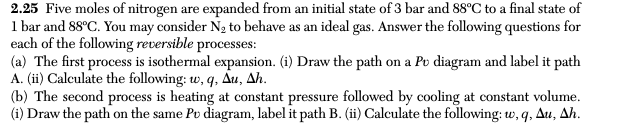# Question Solved1 Answer2.25 Five moles of nitrogen are expanded from an initial state of 3 bar and 88°C to a final state of 1 bar and 88°C. You may consider N, to behave as an ideal gas. Answer the following questions for each of the following reversible processes: (a) The first process is isothermal expansion. (i) Draw the path on a Pu diagram and label it path A. (ii) Calculate the following: w,q, Au, Ah. (b) The second process is heating at constant pressure followed by cooling at constant volume. (i) Draw the path on the same Pu diagram, label it path B. (ii) Calculate the following: w,q, Au, Ah.IZD9FK The Asker · Chemical EngineeringTranscribed Image Text: 2.25 Five moles of nitrogen are expanded from an initial state of 3 bar and 88°C to a final state of 1 bar and 88°C. You may consider N, to behave as an ideal gas. Answer the following questions for each of the following reversible processes: (a) The first process is isothermal expansion. (i) Draw the path on a Pu diagram and label it path A. (ii) Calculate the following: w,q, Au, Ah. (b) The second process is heating at constant pressure followed by cooling at constant volume. (i) Draw the path on the same Pu diagram, label it path B. (ii) Calculate the following: w,q, Au, Ah.
More
Transcribed Image Text: 2.25 Five moles of nitrogen are expanded from an initial state of 3 bar and 88°C to a final state of 1 bar and 88°C. You may consider N, to behave as an ideal gas. Answer the following questions for each of the following reversible processes: (a) The first process is isothermal expansion. (i) Draw the path on a Pu diagram and label it path A. (ii) Calculate the following: w,q, Au, Ah. (b) The second process is heating at constant pressure followed by cooling at constant volume. (i) Draw the path on the same Pu diagram, label it path B. (ii) Calculate the following: w,q, Au, Ah.